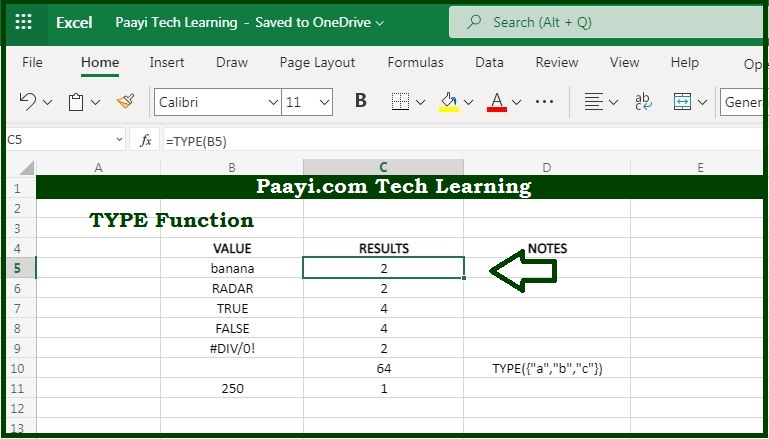# Learn How to Use Microsoft Excel TYPE Function

Written by | 0 Comments | 758 Views

In this article, you will learn how to use the Microsoft Excel TYPE function and its prime function in Microsoft Excel. You will also get to know the Microsoft Excel TYPE function return value and syntax with the help of some examples.

Microsoft Excel TYPE Function

The main use of the Microsoft Excel "TYPE" Function is to get the type of value in the cell. So, you can able to return the numeric code representing "type" in 5 categories, that includes:

number = 1,

text = 2,

logical = 4,

error = 16, and

array = 64.

Use TYPE when the operation of a formula depends on the type of value in a particular cell.

Return Value of TYPE Function

The return value will be the numeric code representing the number.

Syntax of TYPE Function

=TYPE(value)

Where the arguments:

• value: It is the value to check the type of.

## How to Use Microsoft Excel TYPE Function?You can use the TYPE to test the value in a particular cell so that other functions that depend on the type can perform as expected. Below we have provided the table, that represents the possible type codes returned from the TYPE function and the meaning:

 Type Code Meaning 1 Number 2 Text 4 Logical Value 16 Error Value 64 Array

It  should be also noted that:

• With the help of the TYPE function, you can test for formulas because the TYPE function evaluates the result of formulas and functions.
• You can also use the TYPE function to evaluate the type of data that is returned by a function or formula.